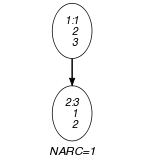## 5.22. allperm

Origin
Constraint

$\mathrm{𝚊𝚕𝚕𝚙𝚎𝚛𝚖}\left(\mathrm{𝙼𝙰𝚃𝚁𝙸𝚇}\right)$

Synonyms

$\mathrm{𝚊𝚕𝚕}_\mathrm{𝚙𝚎𝚛𝚖}$, $\mathrm{𝚊𝚕𝚕}_\mathrm{𝚙𝚎𝚛𝚖𝚞𝚝𝚊𝚝𝚒𝚘𝚗𝚜}$.

Type
 $\mathrm{𝚅𝙴𝙲𝚃𝙾𝚁}$ $\mathrm{𝚌𝚘𝚕𝚕𝚎𝚌𝚝𝚒𝚘𝚗}\left(\mathrm{𝚟𝚊𝚛}-\mathrm{𝚍𝚟𝚊𝚛}\right)$
Argument
 $\mathrm{𝙼𝙰𝚃𝚁𝙸𝚇}$ $\mathrm{𝚌𝚘𝚕𝚕𝚎𝚌𝚝𝚒𝚘𝚗}\left(\mathrm{𝚟𝚎𝚌}-\mathrm{𝚅𝙴𝙲𝚃𝙾𝚁}\right)$
Restrictions
 $|\mathrm{𝚅𝙴𝙲𝚃𝙾𝚁}|\ge 1$ $\mathrm{𝚛𝚎𝚚𝚞𝚒𝚛𝚎𝚍}$$\left(\mathrm{𝚅𝙴𝙲𝚃𝙾𝚁},\mathrm{𝚟𝚊𝚛}\right)$ $\mathrm{𝚛𝚎𝚚𝚞𝚒𝚛𝚎𝚍}$$\left(\mathrm{𝙼𝙰𝚃𝚁𝙸𝚇},\mathrm{𝚟𝚎𝚌}\right)$ $\mathrm{𝚜𝚊𝚖𝚎}_\mathrm{𝚜𝚒𝚣𝚎}$$\left(\mathrm{𝙼𝙰𝚃𝚁𝙸𝚇},\mathrm{𝚟𝚎𝚌}\right)$
Purpose

Given a matrix $ℳ$ of domain variables, enforces that the first row is lexicographically less than or equal to all permutations of all other rows. Note that the components of a given vector of the matrix $ℳ$ may be equal.

Example
$\left(〈\mathrm{𝚟𝚎𝚌}-〈1,2,3〉,\mathrm{𝚟𝚎𝚌}-〈3,1,2〉〉\right)$

The $\mathrm{𝚊𝚕𝚕𝚙𝚎𝚛𝚖}$ constraint holds since vector $〈1,2,3〉$ is lexicographically less than or equal to all the permutations of vector $〈3,1,2〉$ (i.e., $〈1,2,3〉$, $〈1,3,2〉$, $〈2,1,3〉$, $〈2,3,1〉$, $〈3,1,2〉$, $〈3,2,1〉$).

Typical
 $|\mathrm{𝚅𝙴𝙲𝚃𝙾𝚁}|>1$ $|\mathrm{𝙼𝙰𝚃𝚁𝙸𝚇}|>1$
Symmetry

One and the same constant can be added to the $\mathrm{𝚟𝚊𝚛}$ attribute of all items of $\mathrm{𝙼𝙰𝚃𝚁𝙸𝚇}.\mathrm{𝚟𝚎𝚌}$.

Arg. properties

Suffix-contractible wrt. $\mathrm{𝙼𝙰𝚃𝚁𝙸𝚇}.\mathrm{𝚟𝚎𝚌}$ (remove items from same position).

Usage

A symmetry-breaking constraint.

Keywords
Arc input(s)

$\mathrm{𝙼𝙰𝚃𝚁𝙸𝚇}$

Arc generator
$\mathrm{𝐶𝐿𝐼𝑄𝑈𝐸}$$\left(<\right)↦\mathrm{𝚌𝚘𝚕𝚕𝚎𝚌𝚝𝚒𝚘𝚗}\left(\mathrm{𝚖𝚊𝚝𝚛𝚒𝚡}\mathtt{1},\mathrm{𝚖𝚊𝚝𝚛𝚒𝚡}\mathtt{2}\right)$

Arc arity
Arc constraint(s)
 $•\mathrm{𝚖𝚊𝚝𝚛𝚒𝚡}\mathtt{1}.\mathrm{𝚔𝚎𝚢}=1$ $•\mathrm{𝚖𝚊𝚝𝚛𝚒𝚡}\mathtt{2}.\mathrm{𝚔𝚎𝚢}>1$ $•$$\mathrm{𝚕𝚎𝚡}_\mathrm{𝚕𝚎𝚜𝚜𝚎𝚚}_\mathrm{𝚊𝚕𝚕𝚙𝚎𝚛𝚖}$$\left(\mathrm{𝚖𝚊𝚝𝚛𝚒𝚡}\mathtt{1}.\mathrm{𝚟𝚎𝚌},\mathrm{𝚖𝚊𝚝𝚛𝚒𝚡}\mathtt{2}.\mathrm{𝚟𝚎𝚌}\right)$
Graph property(ies)
$\mathrm{𝐍𝐀𝐑𝐂}$$=|\mathrm{𝙼𝙰𝚃𝚁𝙸𝚇}|-1$

Graph class
 $•$$\mathrm{𝙰𝙲𝚈𝙲𝙻𝙸𝙲}$ $•$$\mathrm{𝙱𝙸𝙿𝙰𝚁𝚃𝙸𝚃𝙴}$ $•$$\mathrm{𝙽𝙾}_\mathrm{𝙻𝙾𝙾𝙿}$

Graph model

We generate a graph with an arc constraint $\mathrm{𝚕𝚎𝚡}_\mathrm{𝚕𝚎𝚜𝚜𝚎𝚚}_\mathrm{𝚊𝚕𝚕𝚙𝚎𝚛𝚖}$ between the vertex corresponding to the first item of the $\mathrm{𝙼𝙰𝚃𝚁𝙸𝚇}$ collection and the vertices associated with all other items of the $\mathrm{𝙼𝙰𝚃𝚁𝙸𝚇}$ collection. This is achieved by specifying that (1) an arc should start from the first item (i.e., $\mathrm{𝚖𝚊𝚝𝚛𝚒𝚡}\mathtt{1}.\mathrm{𝚔𝚎𝚢}=1$) and (2) an arc should not end on the first item (i.e., $\mathrm{𝚖𝚊𝚝𝚛𝚒𝚡}\mathtt{2}.\mathrm{𝚔𝚎𝚢}>1$). We finally state that all these arcs should belong to the final graph. Parts (A) and (B) of Figure 5.22.1 respectively show the initial and final graph associated with the Example slot.

##### Figure 5.22.1. Initial and final graph of the $\mathrm{𝚊𝚕𝚕𝚙𝚎𝚛𝚖}$ constraint(a) (b)